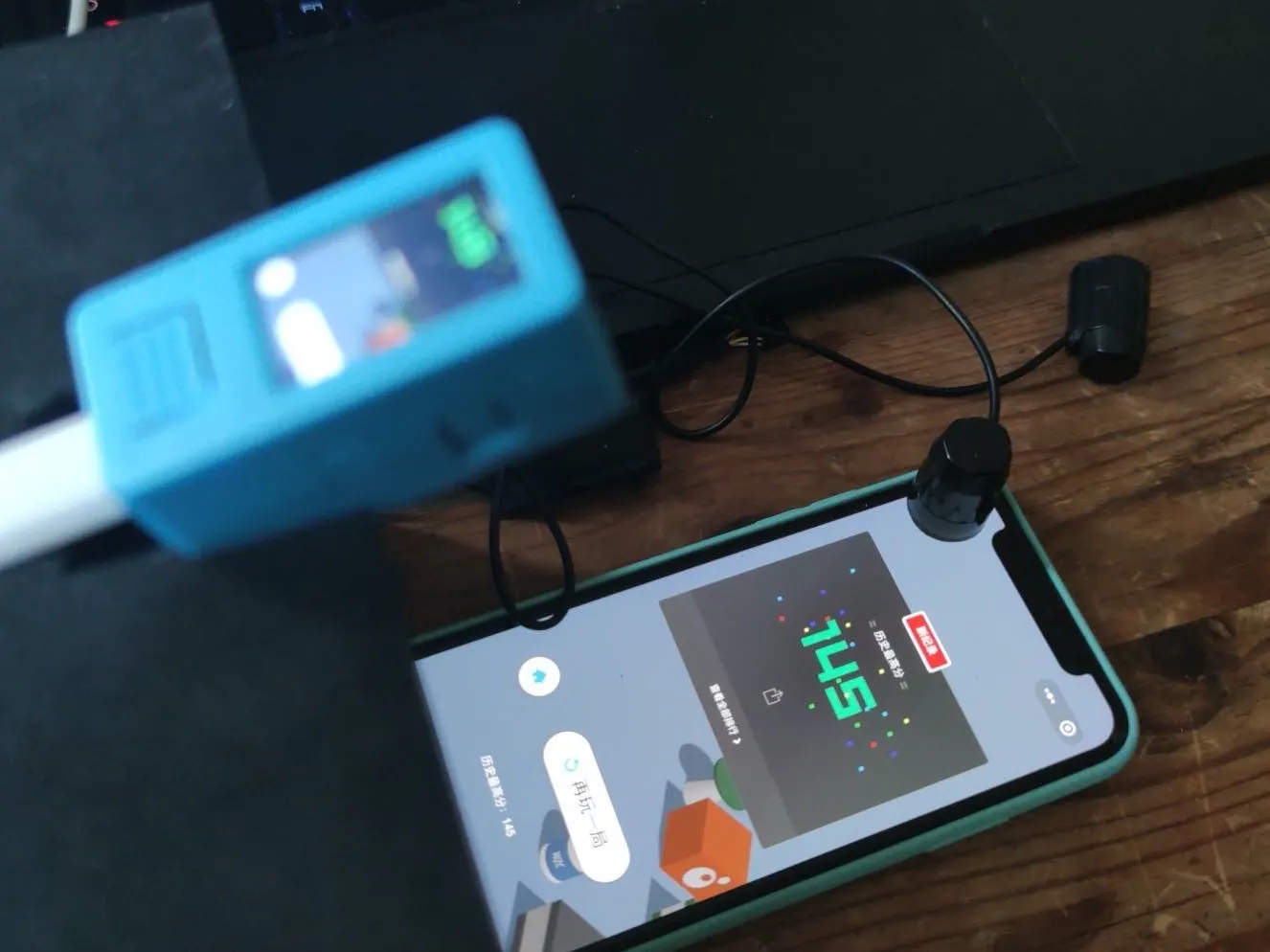# M5StickV deep learning for WeChat Jump game

Thank @沧海 for his contribution We choose wechat jump, a classic little game, as a carrier of target detection test.## Things used in this project

### Hardware components

 M5Stack M5StickV
×1
 Touch screen Clicker
×1

### Software apps and online services

 M5Stack V-Training

## Code

### Autocapture program

Python
```import audio
import gc
import image
import lcd
import sensor
import sys
import time
import uos
import os
#import KPU as kpu
from fpioa_manager import *
from machine import I2C
from Maix import I2S, GPIO

#
# initialize
#
lcd.init()
lcd.rotation(2)

fm.register(board_info.SPK_SD, fm.fpioa.GPIO0)
spk_sd=GPIO(GPIO.GPIO0, GPIO.OUT)
spk_sd.value(1) #Enable the SPK output

fm.register(board_info.SPK_DIN,fm.fpioa.I2S0_OUT_D1)
fm.register(board_info.SPK_BCLK,fm.fpioa.I2S0_SCLK)
fm.register(board_info.SPK_LRCLK,fm.fpioa.I2S0_WS)

wav_dev = I2S(I2S.DEVICE_0)

#fm.register(board_info.BUTTON_A, fm.fpioa.GPIO1)
#but_a=GPIO(GPIO.GPIO1, GPIO.IN, GPIO.PULL_UP) #PULL_UP is required here!

#fm.register(board_info.BUTTON_B, fm.fpioa.GPIO2)
#but_b = GPIO(GPIO.GPIO2, GPIO.IN, GPIO.PULL_UP) #PULL_UP is required here!

currentImage=0

def play_sound(filename):
try:
player = audio.Audio(path = filename)
player.volume(20)
wav_info = player.play_process(wav_dev)
wav_dev.channel_config(wav_dev.CHANNEL_1, I2S.TRANSMITTER,resolution = I2S.RESOLUTION_16_BIT, align_mode = I2S.STANDARD_MODE)
wav_dev.set_sample_rate(wav_info)
spk_sd.value(1)
while True:
ret = player.play()
if ret == None:
break
elif ret==0:
break
player.finish()
spk_sd.value(0)
except:
pass

def initialize_camera():
err_counter = 0
while 1:
try:
sensor.reset() #Reset sensor may failed, let's try some times
break
except:
err_counter = err_counter + 1
if err_counter == 20:
lcd.draw_string(lcd.width()//2-100,lcd.height()//2-4, "Error: Sensor Init Failed", lcd.WHITE, lcd.RED)
time.sleep(0.1)
continue

sensor.set_pixformat(sensor.RGB565)
sensor.set_framesize(sensor.QVGA) #QVGA=320x240
sensor.run(1)

try:
img = image.Image("/sd/startup.jpg")
lcd.display(img)
except:
lcd.draw_string(lcd.width()//2-100,lcd.height()//2-4, "Error: Cannot find start.jpg", lcd.WHITE, lcd.RED)

time.sleep(2)

initialize_camera()

#currentDirectory = 1

if "sd" not in os.listdir("/"):
lcd.draw_string(lcd.width()//2-96,lcd.height()//2-4, "Error: Cannot read SD Card", lcd.WHITE, lcd.RED)

try:
os.mkdir("/sd/train")
except Exception as e:
pass

isButtonPressedA = 0

try:
while(True):
img = sensor.snapshot()
disp_img=img.copy()
disp_img.draw_rectangle(0,60,320,1,color=(0,144,255),thickness=10)
#disp_img.draw_string(50,55,"Train:%03d/35   Class:%02d/10"%(currentImage,currentDirectory),color=(255,255,255),scale=1)
lcd.display(disp_img)

#if but_a.value() == 0 and isButtonPressedA == 0:
img.save("/sd/train/" + str(currentImage) + ".jpg", quality=95)
play_sound("/sd/kacha.wav")
time.sleep(3)  #每隔三秒拍摄一张
currentImage = currentImage + 1
#    isButtonPressedA = 1

#if but_a.value() == 1:
#    isButtonPressedA = 0

except KeyboardInterrupt:
pass
```

### M5StickV Controller

Python
```import image
import lcd
import sensor
import sys
import time
import KPU as kpu
from fpioa_manager import *
import math
import KPU as kpu
from Maix import GPIO
import utime

#使用grove的g35作为继电器执行pin，注册pin以及初始化
fm.register(35,fm.fpioa.GPIOHS0)
relay = GPIO(GPIO.GPIOHS0,GPIO.OUT)

relay.value(0)
#lcd初始化
lcd.init()
lcd.rotation(2)
#载入已训练模型

anchor = (0.33340788 * 16, 0.70065861 * 16, 0.18124964 * 16, 0.38986752 * 16, 0.08497349 * 16, 0.1527057 * 16)

a = kpu.init_yolo2(task, 0.05, 0.05, 3, anchor)
#注：第一个是兴趣框的精确度的阈值
#第二个参数，是目标的精确度的阈值，现在是0.05，调高了就会更可靠，但是可能识别率低
#调低了，容易识别到，但是也同时容易误识别。

sensor.reset()
sensor.set_pixformat(sensor.RGB565)
sensor.set_framesize(sensor.QVGA)
sensor.set_windowing((320, 224))
sensor.run(1)

lcd.clear()

print("Init Done.")

counter = 1 #计数器
code_stake = [] #目标检测集合
minh = 1000 #目标跳台坐标
minh_y = 0
toy_x = 0 #人偶坐标
toy_y = 0
pressed_time = 0.0 #模拟手指的触屏时间

distance_factor = 0.25  #跳跃距离系数，此系数经过与实际手机屏幕校正后，据实际情况使用

while(True):
img = sensor.snapshot()
#print("code....",code）
if code:
if counter < 5: #累计5次识别，将识别结果累加到code_stake[]当中
code_stake = code_stake + code
counter = counter  + 1
else:
counter = 0
minh = 1000
minh_y = 0
toy_x = 0
toy_y = 0
#注：i.rect()   i.rect()    i.rect()    i.rect()   分别是兴趣框的x,y,w,h
for i in code_stake:
if i.classid() == 0:  #识别到跳台,可能镜头里有多个跳台，我们需跳跃的是离摄像头最远的跳台
if minh > i.rect() + i.rect()//2:
minh = i.rect() + i.rect()//2  #计算跳台兴趣框中心点横坐标
minh_y = i.rect() + i.rect()//2#计算跳台兴趣框中心点纵坐标
else: #识别到人偶
toy_x = i.rect() + i.rect()//2 #计算人偶兴趣框中心点横坐标
toy_y = i.rect() + i.rect()//2 #计算人偶兴趣框中心点纵坐标
pressed_time = math.sqrt((minh - toy_x)**2 + (minh_y-toy_y)**2) / 100 * distance_factor #02-25更正，(minh_y-toy_y)
#pressed_time =人偶中心点至跳台中心点距离*跳跃距离系数
img.draw_arrow(toy_x, toy_y, minh, minh_y)  #画人偶至跳台中心点连线，表明跳跃路径
img.draw_string((toy_x+minh)//2, (minh_y+toy_y)//2, "%.03f" % (pressed_time), lcd.BLACK) #显示跳跃参数

for i in code:
if i.classid() == 0: #涉及跳台为红色标记
c = lcd.RED
else:
c = lcd.GREEN #涉及人偶为绿色标记
img.draw_circle(i.rect() + i.rect()//2, i.rect() + i.rect()//2, 3)#目标（跳台或人偶）中心点标记
img.draw_rectangle(i.rect(), i.rect(), i.rect(), i.rect(), c) #标注目标（跳台或人偶）兴趣框
lcd.display(img)

if counter == 0: #5次识别完毕
relay.value(1) #继电器触发，模拟手指触屏动作
utime.sleep_ms(int(pressed_time * 1000)) #经过pressed_time延时
relay.value(0) #继电器接点返回，模拟抬起手指
code_stake = [] #识别集清零
utime.sleep_ms(2000)

else:
lcd.display(img)